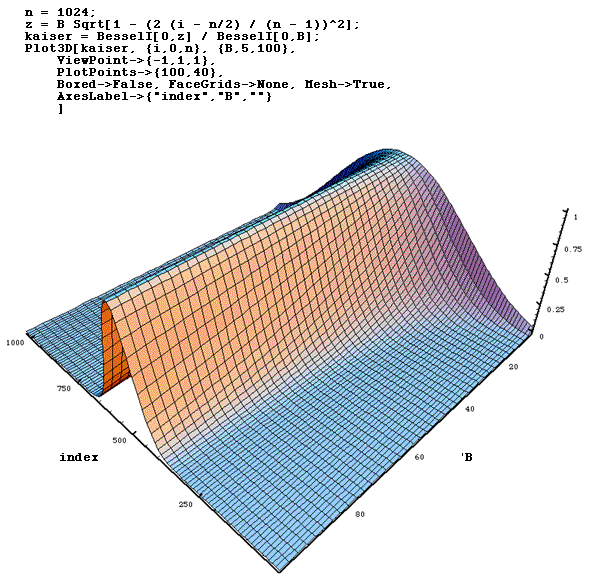# Windows

Welch -- Bartlett -- Hanning/Hamming -- Kaiser

Written by Paul Bourke
August 1998

### Welch

 The Welch window for N points is defined as where 0 <= i < N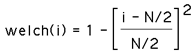Centered around 0 this looks like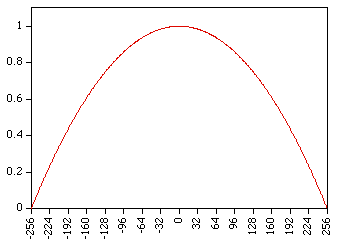Commonly used as a window for power spectral estimation.
The magnitude response is### Bartlett window

 Also known simply as the Triangular window The Bartlett window of width N is defined as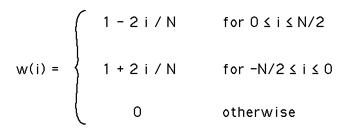The function is simply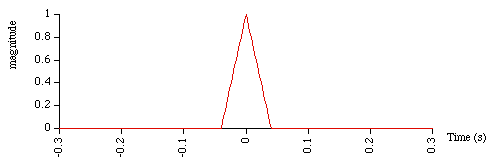The frequency response is sinc2 (a triangle is the convolution of two square windows so the convolution is the product of two sinc functions).### Hanning/Hamming window

 Also known as the raised cosine window The Hanning window for N points is defined as where -N/2 <= i < N/2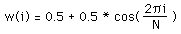The Hamming window is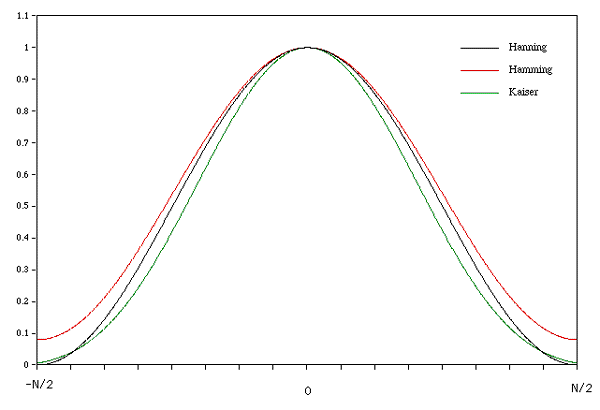These are specific examples from a general family of curves of the form w(i) = a + (1 - a) cos(2 pi i / N)

The magnitude response isShown below is the surface generated in Mathematica of the above function varying the parameter "a".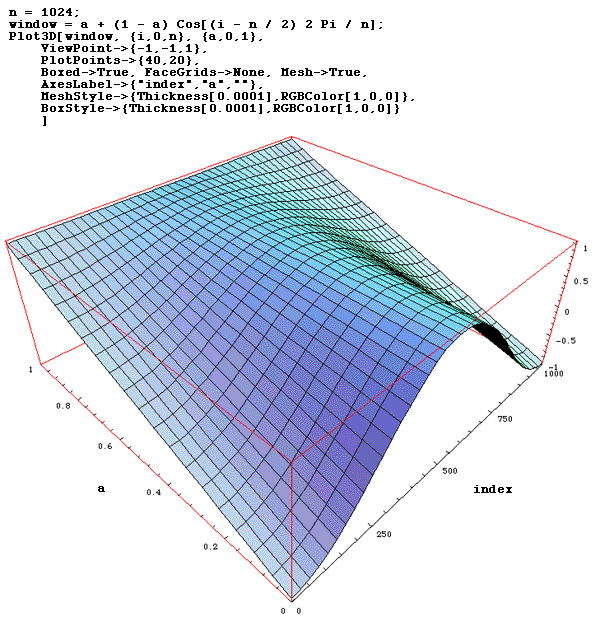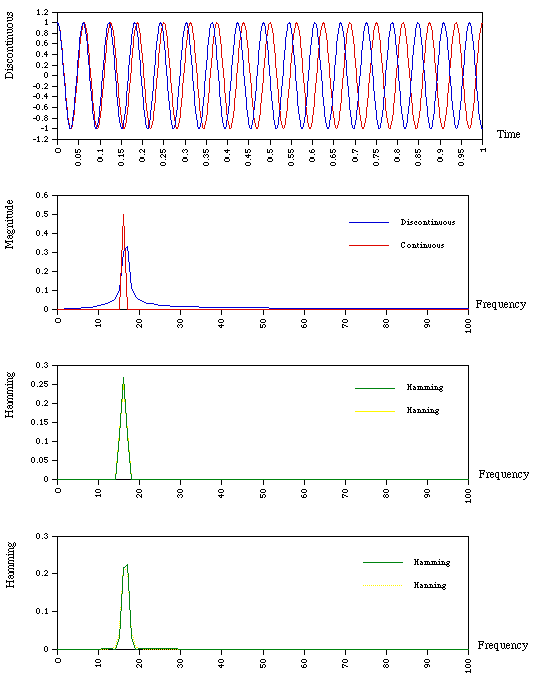### Kaiser-Bessel window

The Kaiser or Kaiser-Bessel window is an approximation to a restricted time duration function with minimum energy outside some specified band. In the discrete case it is defined as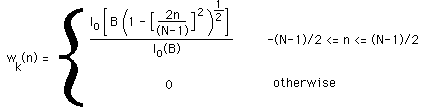where N is the window width, B is half the time-bandwidth product. B determines the trade-off between the magnitude of the side-lobes and the energy in the main lobe and is often specified as a half integer multiple of pi. Io is the zero order modified Bessel function of the first kind, a series expansion of which is: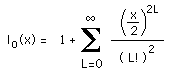The expansion to 20 decimal places isAnalytic forms of the spectrum are not available but it can be shown that the frequency spectrum in the continuous case is proportional to: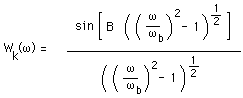where wb is the width of the central lobe.

 Example curves for different values of B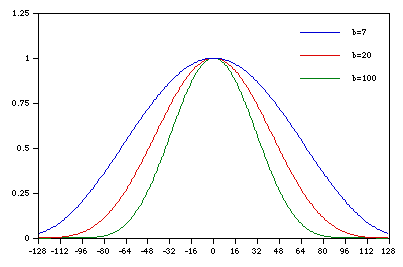Using Mathematics a surface plot showing the window as a function of B.